# Domain invariance

(diff) ← Older revision | Latest revision (diff) | Newer revision → (diff)
Let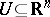be open. Ifis a one-to-one continuous function, then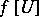is open and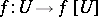is a homeomorphism. This is called the Brouwer invariance of domain theorem, and was proved by L.E.J. Brouwer in [a1]. This result immediately implies that if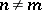, thenand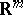are not homeomorphic. A similar result for infinite-dimensional vector spaces does not hold, as the subspace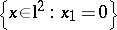ofshows. But Brouwer's theorem can be extended to compact fields in Banach spaces of type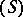, as was shown by J. Schauder [a3]. Here, a compact field (a name coming from "compact vector field" ) is a mapping of the form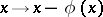, witha compact mapping. A more general result for arbitrary Banach spaces was established (by using degree theory for compact fields) by J. Leray [a2]. Several important results in the theory of differential equations were proved by using domain invariance as a tool.